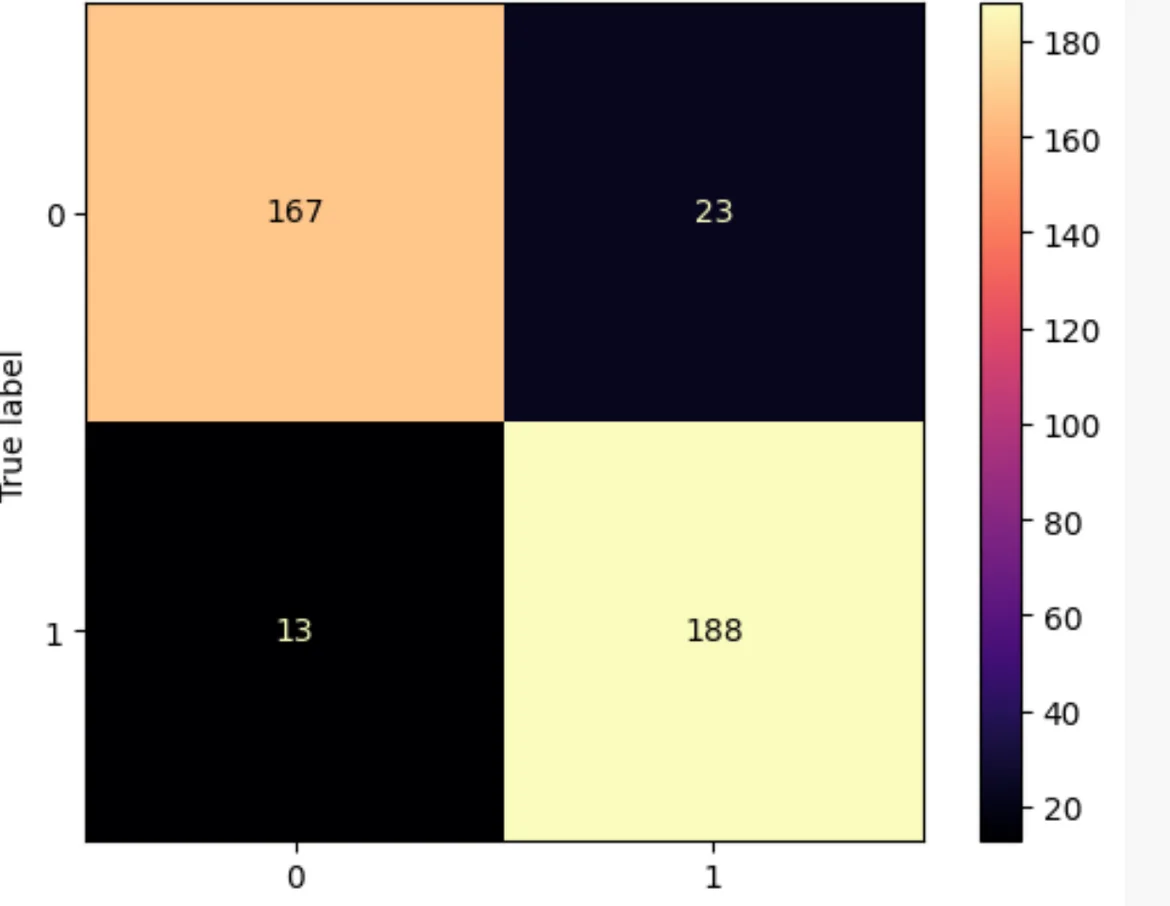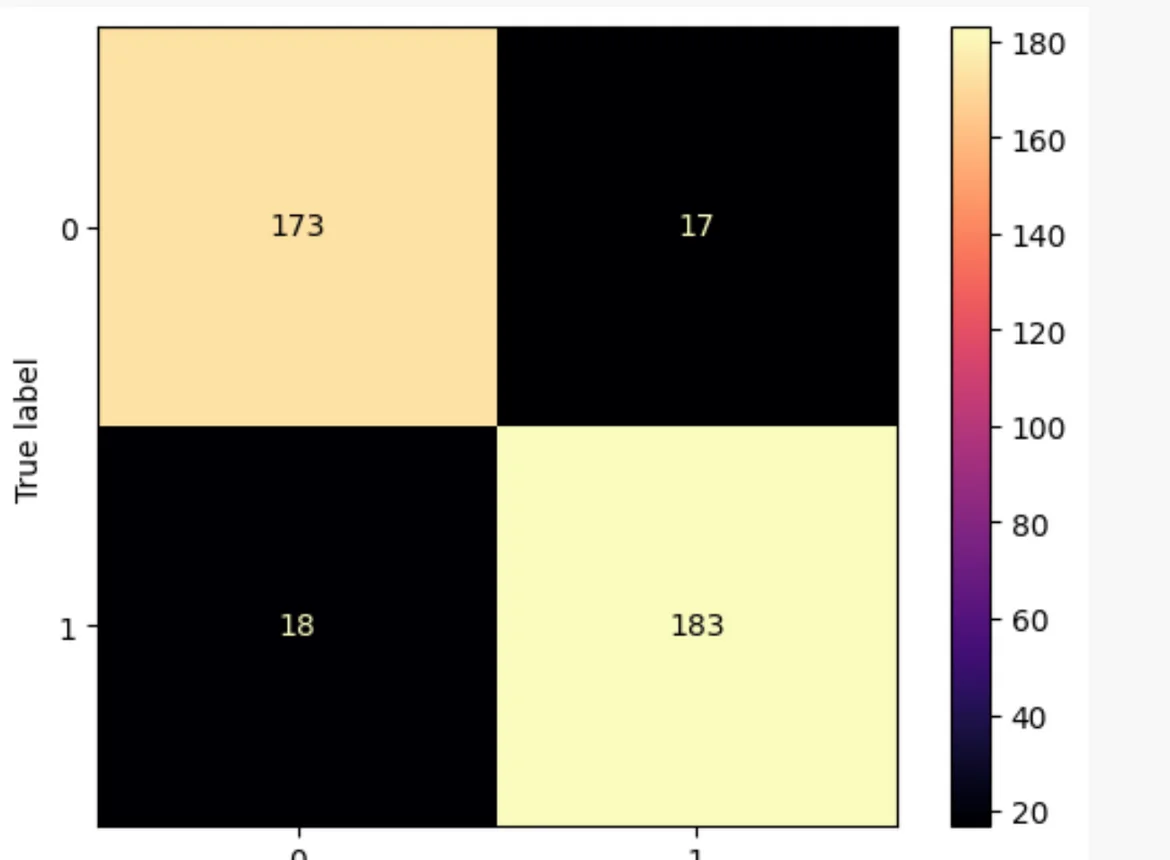31 Aug 2023

Posted on:

09 Aug 2023

0

Performing data engineering before data segmentation is generally recommended to ensure consistent and meaningful feature engineering and preprocessing across both training and testing datasets is that true? I got confuse with the vectorizer method(consequences of transforming the dataset **before** train-test splitting). I got the same output so know i confuse if its better to transform it after or before the data segmentation.

Instructor
Posted on:

09 Aug 2023

1

Hey Gabriela,

Thank you for reaching out and thank you for engaging with the course!

Let me forward you to the thread below where a similar question was posed and answered:

https://365datascience.com/q/6670a91021

Don't hesitate to ask if something has remained unclear.

Kind regards,

365 Hristina

Posted on:

11 Aug 2023

1

Hello, thank you for answering. Now I'm triying to interpret the results.The first screenshot is from my confusion of matrix display with the file transforming the dataset **before** train-test splitting, (173,183) and the second is with the transformation after the train-test- splitting( 167,188). Does this result make sense, performing the transformation before the split is more accurate in this example ?" Thank you, I'm I little bit lose.Posted on:

11 Aug 2023

0

I'm sorry I didn't mention the exercise is the # Multinomial Naïve Bayes Classifier - the YouTube Dataset Thank you

Instructor
Posted on:

14 Aug 2023

0

Hey Gabriela,

Thank you for clarifying!

As you pointed out, the model in which you've applied `CountVectorizer()` before the train-test split (let's call it Model 1) gives a higher accuracy than the model in which `CountVectorizer()` is applied after the splitting (let's call it Model 2). Note that the higher accuracy of Model 1 is misleading. Why is that?

Let's say we implement Model 2 (the correct approach) in which the order of operation is the following:

1. Declare the `inputs` and `target` variables.

2. Split the `inputs `variable into `x_train` and `x_test`.

3. Apply the `fit_transform()` method from `CountVectorizer()` on the `x_train` variable.

4. Apply the `transform()` method from `CountVectorizer()` on the `x_test` variable.

Step 2 of this procedure—splitting the inputs variable—separates the training from the test datasets. This means there might be words in the test set that are not part of the training set.

Now that we have our data split into training and testing sets, we apply the `fit_transform()` method on the training dataset. Fitting is the process of learning the vocabulary in the training dataset. The words from the training dataset are the only ones that will be familiar to the model.

We then proceed to apply the `transform()` method on the test dataset. This method uses the vocabulary previously learned to transform the words from the test dataset. Some words from the test dataset might be unknown to the encoder. That is perfectly normal; our Naïve Bayes model cannot be trained on all spam messages possible. The purpose of a test set is to be unknown to the model. If we test it on a dataset that it was previously trained on, then our model is not truly tested in a 'real life scenario' :) The model’s accuracy that we obtain in the end is honest.

Instructor
Posted on:

14 Aug 2023

0

Let's say we now implement Model 1 (the incorrect approach) in which the order of operation is the following:

1. Declare the `inputs` and `target `variables.

2. Apply the `fit_tranform()` method from `CountVectorizer() `on the inputs variable.

3. Split the `inputs` variable into `x_train` and `x_test`.

In this situation, the encoder learns the vocabulary from the `inputs `variable. This variable is then split into training and testing datasets. But this time, we know all words entering the test dataset. The Naïve Bayes model we’ll later build will therefore have a heads up on what words to expect from the test dataset. This is not how we would want to test the model. If the test dataset is pure, the model should have no information whatsoever about its content.

It now becomes clear why the accuracy of Model 1 is higher than the accuracy of Model 2 – in the former case, the Naïve Bayes model knew what words to expect. In the latter model, on the other hand, the model encountered words that it had not seen before.

I hope this explains the difference between the two cases. I would suggest studying this article by Jason Brownlee who explains the concept of data leakage in a very clear manner:

https://machinelearningmastery.com/data-leakage-machine-learning/

Still, if something has remained unclear, don't hesitate to ask.

Kind regards,

365 Hristina

Posted on:

31 Aug 2023

1

Hello Hristina Hristova, Thank you so much, is clear now :)